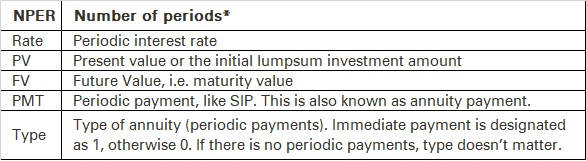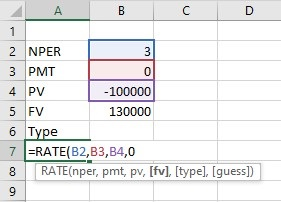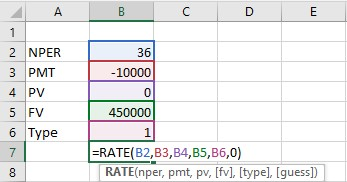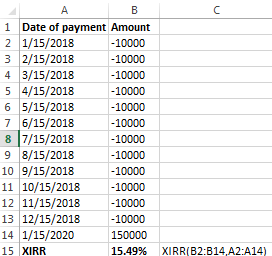Manually calculating returns can be a pain. When there’s an easier option available, why struggle? In this chapter, we’ll look at what formulae to use on Microsoft Excel to calculate mutual fund returns.Return calculation of lumpsum investments through MS Excel

For a lumpsum investment, you will need the following parameters for returns calculations:

You can use RATE function on excel to calculate the annualized rate of return on your lumpsum mutual fund investment. Type the following in an excel cell:*For annualized returns in case of lumpsum investment, investment period will be in number of years. For annualized returns in case of monthly SIP, it will be in number of months.

= RATE (NPER, PMT, PV, FV, TYPE, guess)

• Note: For example, if we want to use the RATE function, we need to type =RATE, choose the RATE function from the suggested functions and press the Tab key.Let’s take an example to understand this calculation better:

If you have invested Rs. 1 lakh in a fund and redeemed it at Rs. 1.3 lakh after 3 years, then,

NPER = Period in number of years = 3 years

PMT = Periodic payment = 0, as there is no periodic investment

PV = Initial investment = - 100,000

FV = 130,000 (No negative sign here as this is an inflow)

Type = It is required only when periodic payments are being made

• Please note that you need to add a negative sign for PV to denote a cash outflow

Guess = Guess is Optional. It is your expected rate of return.Therefore, the rate can be calculated as = RATE(3,0,100000,130000,0) = 9.14% p.a.

## Return calculation of SIP investment through MS Excel

You can use the same Rate function to calculate returns for SIP as well.

For example, assume that you invest Rs. 10,000 p.m. in a fund for 3 years and redeem it at Rs. 4.5 lakh after 3 years.

In this case,

NPER = No. of monthly payments = 3*12 = 36

PMT = Periodic payment or SIP amount = -10, 000

PV = Initial investment = 0 (as there is no lumpsum investment)

FV = 4,50,000

Type = 1

• Again, note that PMT needs a negative sign to indicate an outflowRate can be calculated as =RATE(36,-10000,0,450000,1) = 1.17%

The rate calculated above is not an annual rate, as we have taken everything in the monthly mode. So, the rate calculated above is p.m. i.e. 1.17% p.m.

How does one convert monthly rates into an annualized return rate?

Step 1: Multiply the return by 12 to convert the return into p.a., compounded monthly i.e. =12*1.17 = 14.04% p.a. compounded monthly

Step 2: Apply the EFFECT function to convert the return into p.a. compounded annually i.e. annualized return

= Effect(nominal rate, npery)

Nominal Rate = p.a. rate compounded monthly/quarterly/semiannually etc.

Npery = compounding period in number of years. In case of monthly compounding, it is 12.

So, the annualized rate of return is =EFFECT(14.04%,12) =14.98% p.a.

Return of SIP if payment is not withdrawn immediately after the tenure of the SIP ends

Here, you need to use the XIRR function to calculate returns.

For instance, let’s say you have invested Rs. 10,000 p.m. in a fund for 1 year and complete the term. You redeem the fund after 2 years at Rs. 1.5 lakh.

What will your annualized return be?

In this case, you use the XIRR function. Draw up a cash flow like below. Assuming that your investment period started on January 15, 2018 and continued till December 15, 2018 and you redeemed the fund on January 15, 2020,

XIRR(values, dates, guess)

### Values:

Cash flow (in the picture below, you can see that we have chosen the array form B2:B14 to capture the cash flow). As this is an outflow, we have prefixed a negative sign against all the investment cash flows and no sign before the cash inflow (redemption value).

### Dates:

Cash flow dates. Usually, the format of the dates is MM/DD/YYYY in MS excel. (In the picture, you can see that we have chosen the array A2:A14).

### Guess:

It is optional. It is an estimate of your expected IRR.With the help of the XIRR function, you can calculate the return for any investment, if you know the cash flow amount and dates.

#### Summary

• MS Excel is a great tool to calculate returns on your mutual funds.
• Use the RATE function on MS Excel to calculate annualized returns on lumpsum investments or even SIP investments.

Use the XIRR function on MS Excel to calculate the return on any investment, if you know the cash flow amount and dates.

With this chapter, we come to the end of the advanced course on mutual fund. You should now know how to decode a mutual fund factsheet, calculate the risk and returns for different mutual funds, know the different parameters to choose the right fund for you and also know how to manage your mutual funds portfolio.

Using the basic and advanced course on mutual funds, you can make smart mutual fund investment decisions.

Disclaimer:

ICICI Securities Ltd. ( I-Sec). Registered office of I-Sec is at ICICI Securities Ltd. ICICI Venture House, Appasaheb Marathe Marg, Prabhadevi, Mumbai - 400 025, India, Tel No : 022 - 6807 7100. I-Sec acts as a Composite Corporate agent having registration number –CA0113. PFRDA registration numbers: POP no -05092018. AMFI Regn. No.: ARN-0845. We are distributors for Mutual funds and National Pension Scheme (NPS). Mutual Fund Investments are subject to market risks, read all scheme related documents carefully. Please note, Mutual Fund and NPS related services are not Exchange traded products and I-Sec is just acting as distributor to solicit these products. Please note, Insurance related services are not Exchange traded products and I-Sec is acting as a corporate agent to solicit these products. All disputes with respect to the distribution activity, would not have access to Exchange investor redressal forum or Arbitration mechanism. The contents herein above shall not be considered as an invitation or persuasion to trade or invest. I-Sec and affiliates accept no liabilities for any loss or damage of any kind arising out of any actions taken in reliance thereon. Investments in securities market are subject to market risks, read all the related documents carefully before investing. The contents herein mentioned are solely for informational and educational purpose.